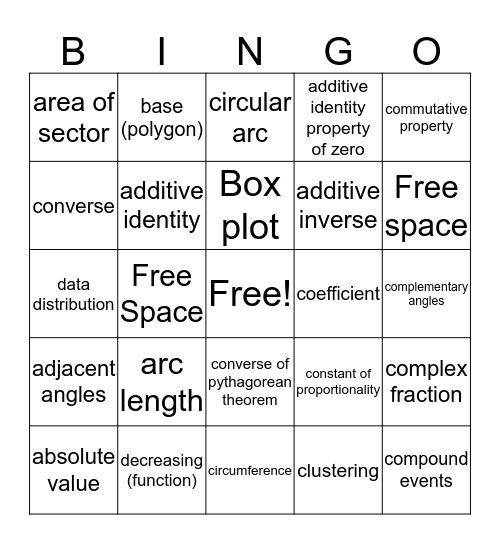# Mathematics Vocab BingoThis bingo card has a free space and 24 words: absolute value, arc length, Box plot, clustering, commutative property, area of sector, base (polygon), circular arc, coefficient, complementary angles, data distribution, additive identity, constant of proportionality, complex fraction, converse, decreasing (function), circumference, additive identity property of zero, Free space, adjacent angles, Free Space, converse of pythagorean theorem, additive inverse and compound events.

## Play Online